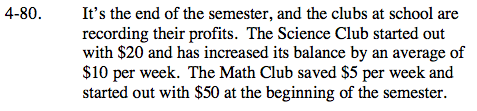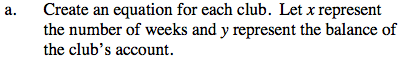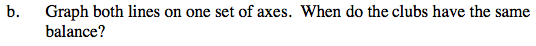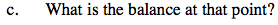### Home > CAAC > Chapter 4 > Lesson 4.2.2 > Problem4-80

4-80.What is the Science Club's starting point?
(y-intercept or b)

How much does their balance increase every week?
(growth rate or m)

Put this into equation (y = mx + b) format.

Follow the same steps to find an equation for the Math Club.What are the x- and y-values of the point where the two lines intersect?